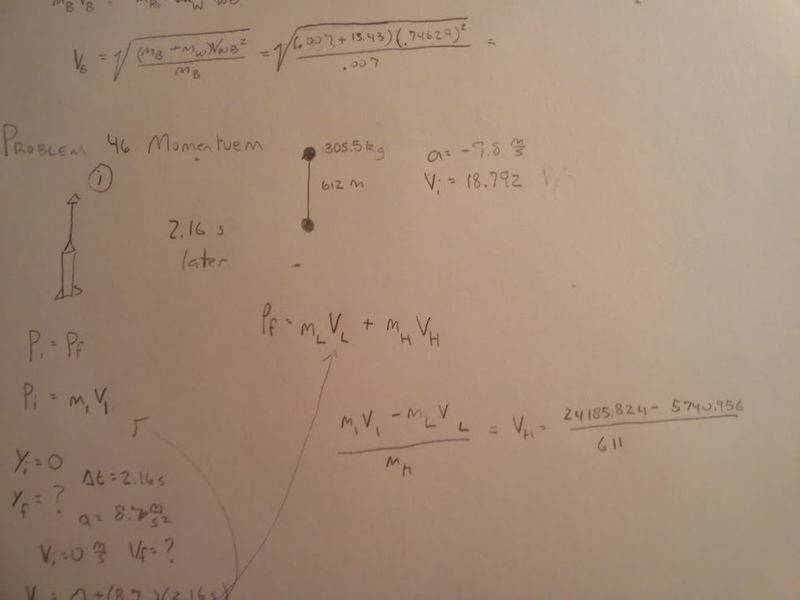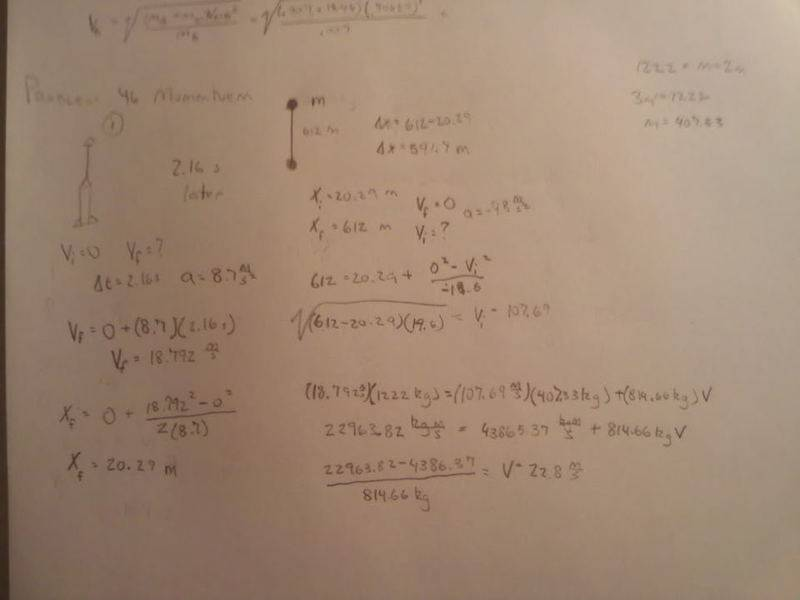# Momentum of rocket exploding

• talaroue
It would be more accurate to think of them as accelerating upward.In summary, the lighter fragment reached a maximum height of 612 m and the heavier fragment had a speed of -25.66 m/s just after the explosion.f

## Homework Statement

A 1222 kg weather rocket accelerates upward at 8.7 m/s2. It explodes 2.16 s after liftoff and breaks into two fragments, one twice as massive as the other. Photos reveal that the lighter fragment traveled straight up and reached a maximum height of 612 m. What was the speed of the heavier fragment just after the explosion

Pi=Pf

## The Attempt at a SolutionYou can calculate the initial speed of the lighter fragment after the explosion from the fact that it travels a distance of (612 m - whatever height the rocket exploded at). After that I'm not sure whether they just want you to get an answer in terms of the mass ratio of the fragments, or whether it is possible to get a numerical answer with the information given.

o i thought the 612 was how high it was for the takeoff. i understand now. and as for the masses would it hurt my anwser if i put those numerical values in? If i do not use those numerical values is it still possible to solve?

You started out ok by solving for the initial velocity of the rocket. You are correct in setting up a momentum equation for just before/after the explosion. I would suggest next calculating the mass of the two pieces. You know two things.

The mass of both pieces = total mass of rocket. (equation 1)
You also know that one piece is twice as large as the other. (equation 2)

Now solve 1) and 2) for the masses.

Now you need to solve for the velocities. You can figure the height of the explosion and are given the maximum height the lighter piece travels. From that you can find what it's speed was right aftr the explosion. All that's left to use momentum to solve for the speed of the bigger piece.

Won't the velocity of the the lighter piece equal the final velocity of the rocket? Why do i need to know the displacement of the lighter piece?

Won't the velocity of the the lighter piece equal the final velocity of the rocket?

No. Think about it. If it did, would momentum be conserved?

You also know that one piece is twice as large as the other. (equation 2)

Yeah, I missed this piece of information the first time I responded. Sorry for any confusion. So in that case it is definitely possible to get a numerical answer.this is my work the second time around but it is wrong again...what did i do wrong?...

Also, it seems like the easiest way to get the initial velocity of the lighter piece would be to use conservation of energy since you know the final PE nd final KE=0 and you know the initial PE, so solve for the initial KE and thus initial velocity.

so the way i found the Vf is wrong?

EDIT: It comes up the same either way.

so where did i go wrong

On your last line you left out a "5" in the number 4386"5".37

You should get that the heavier piece has negative velocity, or that it is traveling downward.

I don't see how though. all my work seems to be right and how do you know that it is traveling down?

I don't see how though. all my work seems to be right and how do you know that it is traveling down?

Your work does seem to be right, but you have made a math mistake in your last line. You have the number 4386.37 in the numerator. But look at your previous line, it should be 43865.37. You have left out the 5 digit.

Put the 5 digit back in and you get a negative number meaning down.

ahhhh... i see that i get a negative number now, but that negative number is wrong now as well.

What answer did you get and what do you think the answer should be?

I got -25.66 m/s...I think that it should be around -50 m/s be right considering the smaller one took off at an extremely fast speed, so the second one with a heavier mass would take off significantly slower.

Your answer seems correct to me, but you logic is a bit faulty. If two masses were sitting still when it exploded then yes the velocities would in the same ratio as the masses, i.e. the bigger one half the speed of the smaller one. However they weren't standing still when it exploded that were both traveling upward. The initial upward speed changes things so that the velocities are not a simple ratio. Some of the initial upward speed will add to the speed of the lighter one and some of the initial speed will subtract from the heavier one that is falling.

However, it's not good to think of this problem as adding/subtraction speeds, it is momentum that is being added/subtracted.

i get it, do you think that since I found the speed of the rocket going up, i should incorporate that into the heavier one going down?

No, that's already accounted for in the momentum equation. Remember that you used the velocity and the mass of the rocket going up on one side of the momentum equation.

o ok, I am just trying to figure out why it isn't coming out correct.